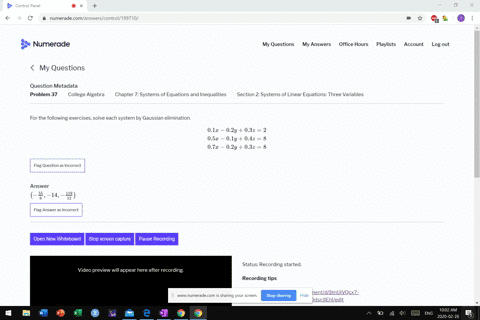Enroll in one of our FREE online STEM bootcamps. Join today and start acing your classes!View Bootcamps03:26
MB
Problem 36

# For the following exercises, solve each system by Gaussian elimination.$$\begin{array}{l}{\frac{1}{40} x+\frac{1}{60} y+\frac{1}{80} z=\frac{1}{100}} \\ {-\frac{1}{2} x-\frac{1}{3} y-\frac{1}{4} z=-\frac{1}{5}} \\ {\frac{3}{8} x+\frac{3}{12} y+\frac{3}{16} z=\frac{3}{20}}\end{array}$$

## Discussion

You must be signed in to discuss.

## Video Transcript

all right. 36 you will notice about this is that there's a a lot of fractions in here, and many have been that not been simplified, for instance. Look at all these denominators here in the first equation. Um, they're all multiples of 10. So basically, what I can do is I can divide multiply this equation by 10. And what that would do with base to get rid of this times 10. Were that this time thinking that this time that this time I'm spending with that. Okay, that simplifies my work. Just a little bit Maur for this one. Now, let me see the last one. It looks like these are all, um, multiples of four, almost four. So I can rewrite this one also, um, so I'm gonna multiply everything by Ford. This is gonna be three halves. That's this is me. This will be 3/3. 3/3. Why? Says what? And then this turns force and three Force Z equals three. Okay. And then I don't need this one anymore. All right, so now let's clear these things of any fractions that we have been through this number that 468 10 um But in fact, he was a role even even so, I can buy them all bite too. So let's do that. Most buy this. Buy two. Get 1/2 despite to you there this or Oh, this bike to you. Yeah. Um Oh, wow. Check this out. This is great. So these aren't the same equation, Okay? Only difference here is that these are all positives on negatives. If I smoke like this one, make them all positive. He's in the same plane in order yet a unique solution. You have to be at three unique planes. There's no no unique solution to this problem, so yeah.